# Containers in C++

## Containers in C++ with Examples:

In this article, I am going to discuss Containers in C++ with Examples. Please read our previous article where we discussed STL Classes in C++ with Examples.

##### Containers in C++:

Containers are the objects that handle a collection of other objects or elements and these implement a well-defined data structure. You must be familiar with some of the popular containers i.e. vectors, stacks, queues, and so on. It is very difficult to write effective code without the use of some of these containers. So, let us see a broad classification of these containers.

##### Classification of Containers in C++: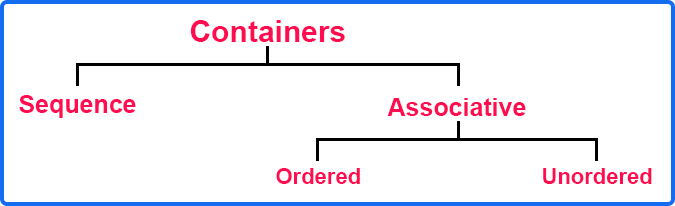At higher levels, we can classify containers into Sequence containers and Associative Containers. And Associative containers can further be classified into ordered and unordered containers. So first, let us see sequence containers.

##### Sequence Containers in C++:

Here, the storage of data elements is done inside these containers. Whereas in associative containers, these implement associative arrays which have a notion of pairs where there is a key and one actual value. We are interested in the actual value but we access them or store them with the use of some key and you can argue that in sets, we do not have values but we have keys. So there the data itself acts as the key and in the map case we have key and value pairs.

Some examples would be vectors, stacks, queues, lists, double-ended queues (deque), and arrays. There is a difference between std::array and std::vector. The array is fixed-sized and the vector is dynamically sized. Now let us see associative containers.

##### Associative Containers in C++:

These are the containers that implement associative arrays. There is a notion of key and actual data. In sequence containers, we store or access elements with the help of their position or index but in the case of associative containers, we store as well as fetch the store data with the help of a key. So, these can be ordered and unordered. So, the difference lies in their underlying implementations.

In ordered cases, there is a notion or order and these are implemented with the help of balanced binary search trees. When you do an in-ordered traversal of a balanced binary search tree then we see that elements are printed in ascending order. And if the tree is a balanced binary search tree, then if it has n elements then the height will be of the order of log n. In binary search trees, all the different operations are of the order of height.

In unordered cases, these are implemented using hash tables so the order is not guaranteed. Here, the expected operation time is in order of 1 i.e. O (1) for most of the operations. In the ordered case, we have set, map, multi-map, and multi-set. Sets and Maps do not allow duplicate keys but multi-map and multi-set allow duplicate keys. So, you can classify it into a singular version and a multi-version. But we would rather recommend ordered and unordered since the underlying implementation is totally different for these two. In unordered cases, we have set, map, multi-map, and multi-set but we write them as an unordered set, unordered map, unordered multi-set, and unordered multi-map. These are implemented using hash tables. In sets and multi-sets, we do not have an associated value with the key. The value is itself acting as the key. In the map, multi-map, unordered map, and unordered multi-map, we have a key to a corresponding value.

Now let us see about these containers in some brief way. Let us give you a brief overview of all these different containers. The first one is the vector.

##### Vector in C++:

It is one of the most popular and widely used containers. Vector implements dynamic size array. Initially, you can just create a vector of int,

std::vector<int> v;

As you keep inserting in v, its size will be increased. It may start with a size of let us say 4. Once you do 4 insertions, it may allocate a new array of size 8.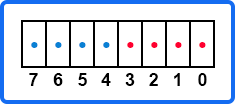It will copy the first 4 elements in the blue portion so that the next 4 push operations will be done within this in constant time. And once this is full it will create another array of double size. Just double the size is one implementation. It can be different on different distributions. When you call the size function then it will show the number of elements that we have inserted. But there is an implicit capacity here (the red portion in the above diagram). So, these are dynamic-sized arrays. This support random access just like an array.

We can write v, v, and so on. So, we can directly jump on any element in constant time. This random access is supported here. Insertion and deletion of elements are supported and elements are stored contiguously in memory. There is one difference from the linked list where you have to follow the stored pointer to find the next location but here elements are stored contiguously. We can increment or decrement the index the same as arrays. So, these are the few things that you should keep in mind. Now the next container is list.

##### List in C++:

This is the implementation of a doubly linked list. An example of a doubly linked list is,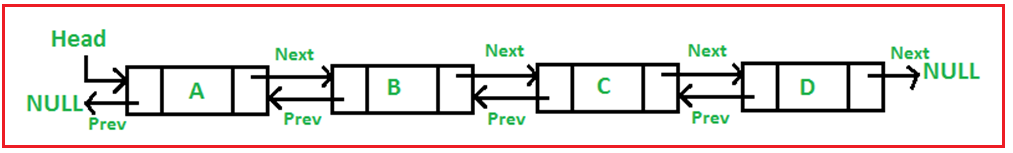Here one node has two pointers. The first is for pointing to the next node and the second is for pointing to the previous node. So, what is the advantage of two-pointers? Let us say you want to insert or delete from the middle. In the case of vector, everything else needs to be shifted that is considered an order of n time i.e. O(n).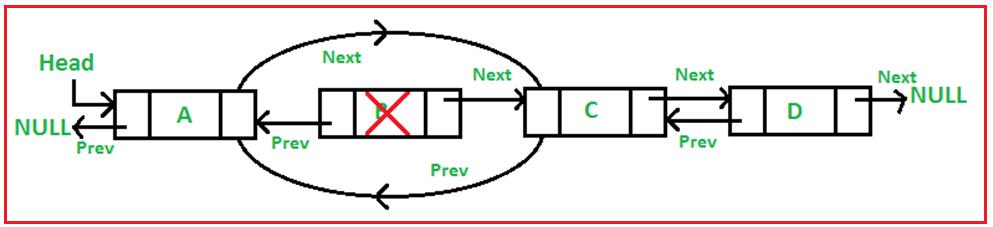In the case of a doubly linked list, when you delete the node i.e. B then the previous node (A) next pointer should be pointed next to the next node that is C and the previous pointer of C should be pointed previously to the previous node that is A. And now clean the memory so that the B node will not be there. So we have deleted a node in O(1) time. So, it does not support random access. You cannot access the node like the way we access arrays or vectors. And here elements are not stored contiguously. So, it will give a different performance than the vector depending on the use case.

There is another version of the list in C++ that we say it a forward list. This is a simple list. You will use,

std::list<int> l;

In angle braces, you can give any data type whether it is primitive or user-defined. The forward list is the implementation of a singly linked list.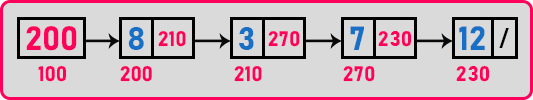So only the next pointer is here. The next container is Deque.

##### Deque in C++:

It is the implementation of a double-ended queue. A double-ended queue means you can insert or remove from any side in your queue. A normal queue is where you can insert from one end and remove from another end.

###### Queue: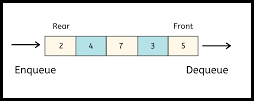###### Deque: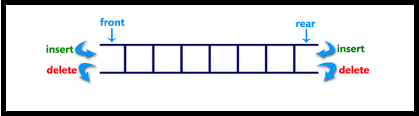It supports push and pop operations at both ends. Elements are not all stored contiguously in memory but rather these are implemented as chunks of continuous data. So, let us say you have a deque of 1000 elements. So maybe 100 elements are contiguous and then the next 100 are at some different locations. There may be different implementations. You can think of its performance somewhere between the vectors and the lists. Now let’s see container adapters.

These are the interfaces that implement special functionality on top of sequence containers. Let us say you have a deque. A deque supports push and pops at both ends. Let us say you want to use its functionality to implement another interface for a stack. So, in a stack, we know that we can push at descend and also pop from the same end. So, at the same end, we have to use push and pop. So, in the front of the deque, we will use the same functionality that is push and pop functions. Then it will now act as a stack. Because we are pushing at one end. When we will pop immediately pushed elements. So, it will be converted into LIFO. So here we are using the functionality of deque to act as another container called a stack. The interface for the stack is different. Stack just exposes push and pop operations whereas deque will expose push front, pop front, push back, and pop back. This is acting as a container adapter. The stack supports push and pops at one end. The ideal choice for the underlying container is there can be different containers using which you can implement stack.

In a queue, you insert at one end and remove from another end. So again, you can use a deque. It supports all four operations i.e. push front, pop front, push back, and pop back. So, it can act like a queue. Deque is a natural choice but you can use vector and list also.

In a priority queue, it supports the operation of a queue but one thing is that it always dequeues the maximum element or rather element with the highest priority, not the maximum. So naturally, it is performed by an array-based data structure called the heap. The underlying container is usually a vector. So, the default would be vector. You can leave it if you are using a priority queue. Here you can use a vector as a content adapter. All these are undying container options because container adapters are templates too. Now let us look at some of the associative containers. So the first one is set.

##### Set in C++:

We are discussing ordered and unordered sets. These are models of the mathematical sets. In mathematics, we have a set in which we are not allowed to have duplicates. So, it is a collection of unique elements that serve as keys. All the associative containers will have some key. Ordered sets are implemented using balanced binary search trees and unordered versions are implemented using hash tables. There are also multi-sets and multi-unordered sets. In multi-sets, the keys are not unique. We can have multiple or duplicate keys. So, we have four possibilities that are set, unordered set, multi-set, and multi-unordered set.

##### Map in C++:

In map also, we have 4 possibilities, map, unordered map, multi-map, and multi-unordered map. These are set of pairs, {(k1,v1), (k2, v2), (k3, v3)}. Here we will be using the keys for all the storing and accessing the values. But actual data will be stored in the value. So, these are key-value pairs. Pair by pair means we have two tuples i.e. (x, y). The first one is key and the second one is value.